home

# How many grains are needed for a provenance study?

The general equation for the calculation of the probability p that at least one of M fractions >= f of the population is missed by all k grains of a sample is: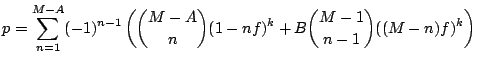(1)
with: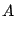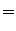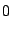if: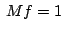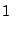if: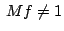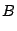if: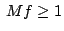if: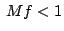Without specifying the number of fractions, the worst case probability pmax becomes: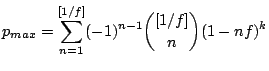(2)

For the following calculators to work, JavaScript must be enabled in your web browser.

1. ## Calculating k as a function of the desired p and f:

Example: to reduce the chance that at least one fraction f >= 0.05 has been missed to less than p = 5%, k = 117 grains have to be dated.
 p: %, f: , k:
2. ## Calculating fact for a given p and k:

Example: if k = 60 grains have been dated, the fraction that we know with (100-p) = 95% certainty not to have missed, is fact = 0.086
 k: , p: %, fact:
3. ## Calculating pmax as a function of k and f:

Example: when k = 60 grains are dated of a uniformly distributed population, the chance that at least one fraction f >= 0.05 has been missed is pmax= 64%.
 k: , f: , pmax %
4. ## Calculating Mopt for a given p, f and k:

Example: if we want to reduce the chance that at least one fraction f >= 0.05 was missed of a uniform population to less than p = 5%, and we have dated k = 100 grains, then we can only use Mopt = 9 bins in the age-histogram.
 p: %, f: , k: , Mopt: